# Contraction of a Lie algebra

An operation inverse to deformation of a Lie algebra. Letbe a finite-dimensional real Lie algebra, let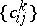be its set of structure constants with respect to a fixed basis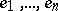and let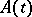,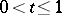, be a curve in the group of non-singular linear transformations ofsuch that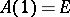. Let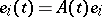and letbe the structure constants ofwith respect to the basis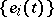. If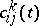tends to some limit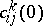as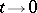, then the algebra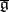defined by these constants relative to the original basis is called a contraction of the initial algebra. The contractionis also a Lie algebra, moreovercan be obtained by means of a deformation of. Ifis the Lie algebra of a Lie group, then the Lie group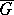corresponding tois called a contraction of the group.

Although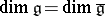, in general these algebras are not isomorphic. For example, if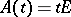, then, so for this contraction the limit algebra is always commutative. The natural generalization of this example is the following: Letbe a subalgebra in, letbe a subspace complementary to, let, moreover,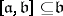and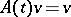for each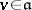,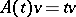for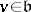. Then in the limitbecomes a commutative ideal of, while at the same time multiplication in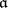and the operation ofonremain the same.

In particular, letbe the Lorentz group,its Lie algebra andthe subalgebra corresponding to the subgroup of rotations of-dimensional space. Then the described contraction ofgives the Lie algebra of the Galilean group(see Galilean transformation; Lorentz transformation). Hence the Lorentz algebra is a deformation of the Galilean algebra, and it can be shown that the complexification of the Galilean algebra has no other deformations; in the real case the Galilean algebra can also be a contraction of the orthogonal Lie algebra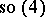. An equivalent method of obtaining the Galilean algebra from the Lorentz algebra is to define the Lorentz algebra as the algebra preserving the Minkowski form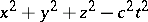, and then letting the velocity of light tend to. As long as, the algebra arising is isomorphic to. Analogously, deforming the Poincaré algebra (the inhomogeneous Lorentz algebra), it is possible to obtain the de Sitter algebrasandof motions of a space of constant curvature. Correspondingly, setting the curvature to 0, one obtains the Poincaré group as a contraction of the de Sitter group.

The connection between these algebras can be extended to representations. If, as in the described examples, there is a matrix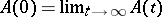, then each representationofgenerates a representation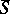of the contraction algebra by the formula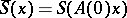for any. The inverse operation (deformation of a representation) is not possible, in general.

How to Cite This Entry:
Contraction of a Lie algebra. A.K. Tolpygo (originator), Encyclopedia of Mathematics. URL: http://encyclopediaofmath.org/index.php?title=Contraction_of_a_Lie_algebra&oldid=12965
This text originally appeared in Encyclopedia of Mathematics - ISBN 1402006098